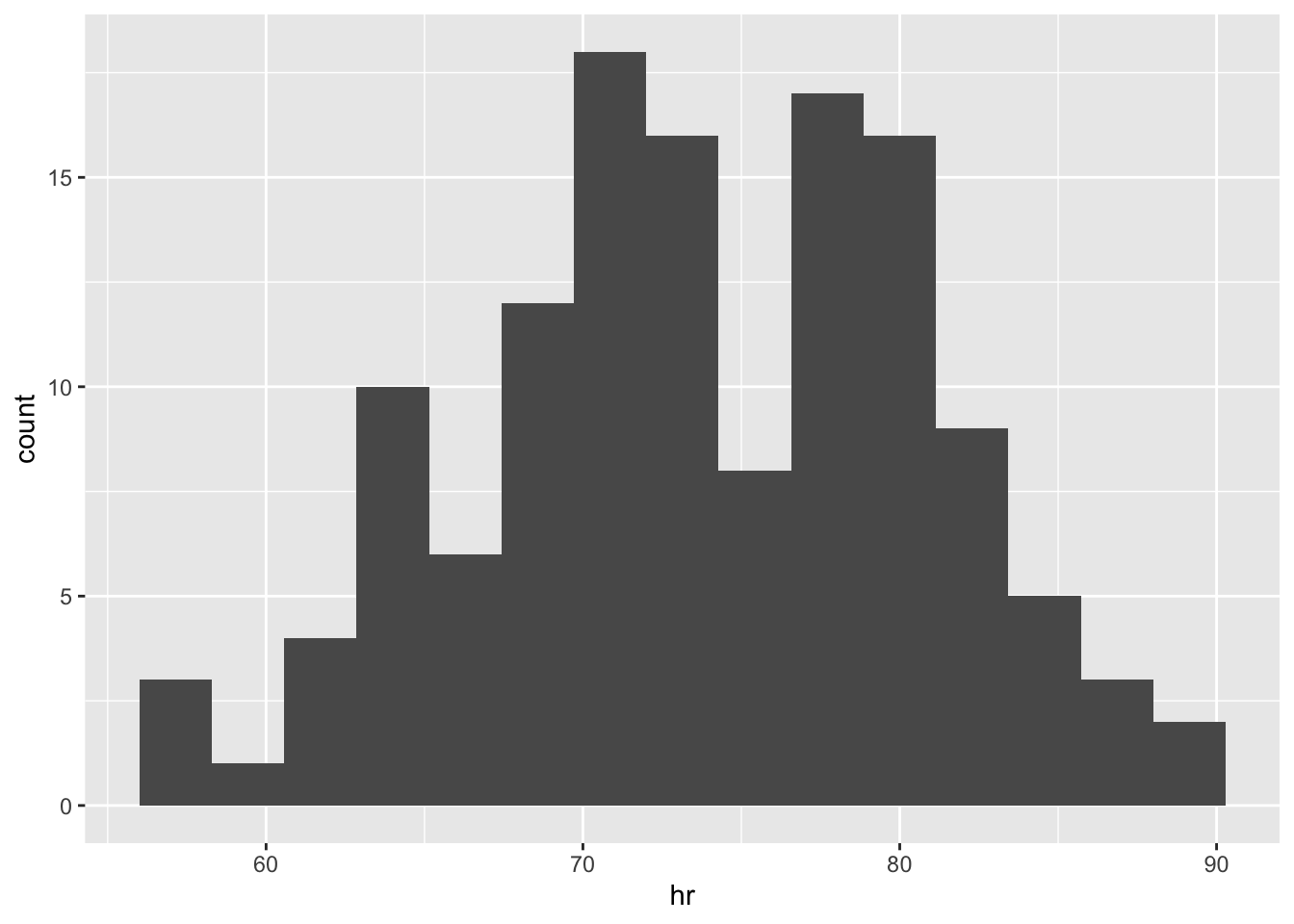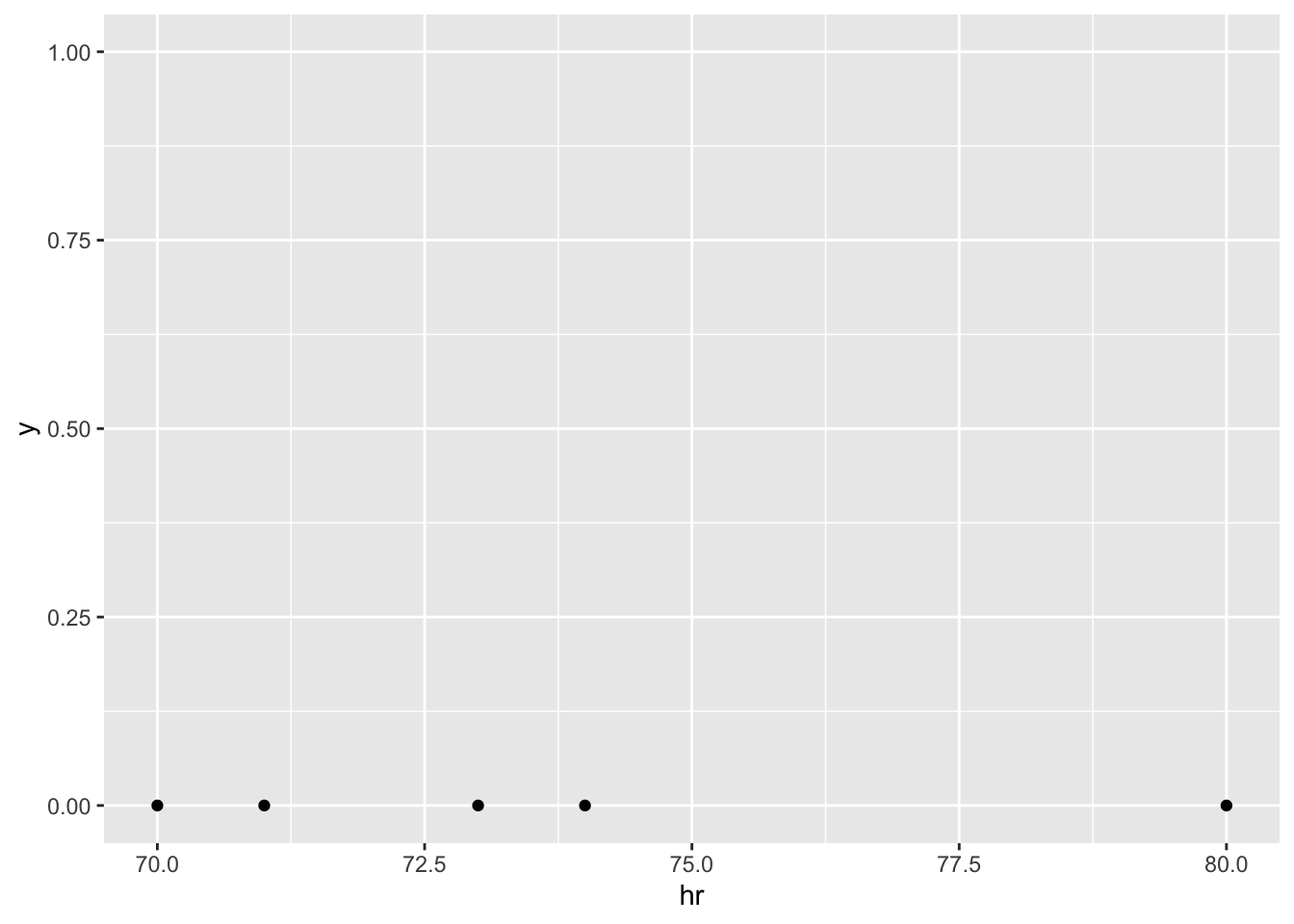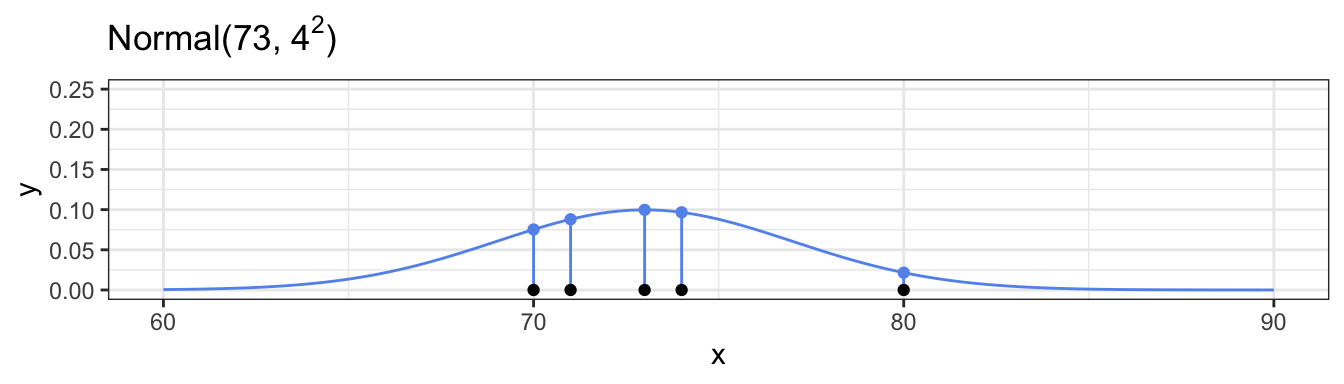## Pulse Rates

We have a data set with the pulses (heart rate, beats per minute) for 130 subjects. Can we fit a normal distribution to these values?

head(pulses)
## # A tibble: 6 x 3
##    temp sex      hr
##   <dbl> <chr> <dbl>
## 1  96.3 Male     70
## 2  96.7 Male     71
## 3  96.9 Male     74
## 4  97   Male     80
## 5  97.1 Male     73
## 6  97.1 Male     75
ggplot(data = pulses, mapping = aes(x = hr)) +
geom_histogram(bins = 15)To make the plots easier to think about, suppose we only had 5 subjects.

pulses_5 <- pulses %>% slice(1:5) %>% arrange(hr)
pulses_5
## # A tibble: 5 x 3
##    temp sex      hr
##   <dbl> <chr> <dbl>
## 1  96.3 Male     70
## 2  96.7 Male     71
## 3  97.1 Male     73
## 4  96.9 Male     74
## 5  97   Male     80
ggplot(data = pulses_5) +
geom_point(mapping = aes(x = hr, y = 0)) +
ylim(c(0, 1))### Our Model

Let $$X_i$$ be the heart rate for subject number $$i$$. We adopt the model that these random variables are i.i.d. with

$$X_i \sim \text{Normal}(\mu, \sigma)$$

### Joint Probability Density if $$X_i \sim \text{Normal}(73, 4^2)$$Individual probability densities are calculated as:

$f(x_i | \mu = 73, \sigma = 4) = (2 \pi 4^2)^{\frac{-1}{2}} \exp\left[ \frac{-1}{2 \cdot 4^2} (x_i - 73)^2 \right]$

For example, our first observation has $$x_1 = 70$$:

$f(70 | \mu = 73, \sigma = 4) = (2 \pi 4^2)^{\frac{-1}{2}} \exp\left[ \frac{-1}{2 \cdot 4^2} (70 - 73)^2 \right]$

In R, for all 5 observations this is calculated as:

dnorm(pulses_5$hr, mean = 73, sd = 4) ##  0.07528436 0.08801633 0.09973557 0.09666703 0.02156933 The joint probability density is calculated as: $f(x_1, x_2, x_3, x_4, x_5 | \mu = 73, \sigma = 4) = \prod_{i = 1}^5f(x_i | \mu = 73, \sigma = 4) = \prod_{i = 1}^5 (2 \pi 4^2)^{\frac{-1}{2}} \exp\left[ \frac{-1}{2 \cdot 4^2} (x_i - 73)^2 \right]$ In R, this is calculated as: dnorm(pulses_5$hr, mean = 73, sd = 4) %>% prod()
##  1.377949e-06

### Joint Probability Density for 3 Candidate Sets of Parameter Values

Parameter Set 1: $$X_i \sim \text{Normal}(73, 4^2)$$

Parameter Set 2: $$X_i \sim \text{Normal}(73, 2^2)$$

Parameter Set 3: $$X_i \sim \text{Normal}(76, 4^2)$$